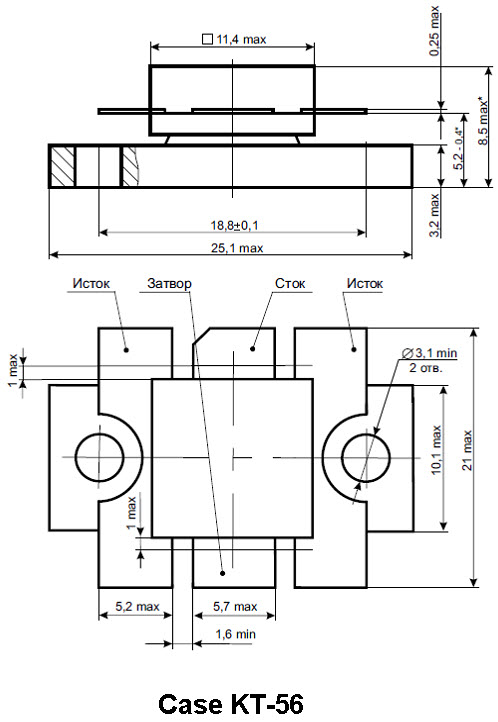# KP979A - Silicon MOS N-Channel Microwave Power Transistor 60 W, up to 230 MHz, Enhancement ModeThe silicon MOS transistor is designed for professional transmitter applications in the HF/VHF frequency range.

Features:

• Performance at 230 MHz, 28 Vdc
• Power Gain: 14 dB Min
• Output Power: 60 W
• Efficiency: 50 % Min
Send request
Send request

## Absolute Maximum Ratings

Drain-Source Voltage VDSS 65 VDC
Gate-Source Voltage VGS ±20 VDC
Operation Junction Temperature Tj -65 ÷ +200 ºС
Storage Temperature Range TSTG -65 ÷ +150 ºC
Thermal Resistance, Junction to Case RqJC 1.45 ºC/W
Total Power Dissipation PD 120 W

## Parameters

ParameterSymbolMin.Typ.Max.Unit
Drain-Source Breakdown Voltage (IDS=20 mA, VGS=0 V) V(BR)DSS 65 VDC
Gate-Source Leakage Current (VGS=20 V, VDS=0 V) IGSS 1 mADC
Zero Gate Voltage Drain Leakage Current (VDS = 28 V, VGS=0 V) IDSS 6 mADC
Gate Threshold Voltage (VDS = 10 V, ID = 50mA) VGS(TH) 2 5 VDC
Forward Transconductance (VDS = 10 V, ID = 3 A) GFS 2 2.2 mhos
Input Capacitance (VDS = 28 V, VGS=0 V, f = 1 MHz) CISS 100 pF
Output Capacitance (VDS = 28 V, VGS=0 V, f = 1 MHz) COSS 90 pF
Reverse Transfer Capacitance (VDS = 28 V, VGS=0 V, f = 1 MHz) CRSS 9 pF
Power Gain (VDS = 28 V, POUT = 60 W, lDQ. = 100 mA,  f = 230 MHz) Gp 14 15 dB
Drain Efficiency (VDS = 28 V, POUT = 60 W, lDQ. = 100 mA,  f = 230 MHz) hD 50 60 %